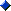Absolute Poker BonusUltimate Bet BonusFull Tilt BonusDoyles Room Bonus

# Poker Article: Texas Holdem Miscellaneous Probabilities

The following Texas Holdem miscellaneous probabilities are used by the top mathematically-inclined poker players currently on tour. Making use of statistical probabilities during the course of a hand, can help you gauge your level of play at a particular juncture. The following odds can be committed to memory, and be incorporated into your game play, to help maximize profits.

• The probability of an opponent having a set of two cards that are paired on the board, when there are 5 total players in the hand, is approximately 43 %.

• The probability of an opponent having a set of two cards that are paired on the board, when there are 4 total players in the hand, is approximately 34 %.

• The probability of an opponent having a set of two cards that are paired on the board, when there are 3 total players in the hand, is approximately 26 %.

• The probability of an opponent having a set of two cards that are paired on the board, when there are total 2 players in the hand, is approximately 17 %.

• The probability of having a gutshot straight draw improve to a straight by the river is approximately 17 %.

• The probability of making a flush by the river when flopping three cards to a flush (that is, making a backdoor flush, known as a runner-runner) is approximately 4 %.

• The probability of another player making a straight by the river when flopping three cards to a straight (that is, making a backdoor straight, known as a runner-runner) is approximately 1.5 %.

• The probability of another player getting an Ace on the turn or river while holding one in your hand and missing on the flop is approximately 13 %.

• The probability of an opponent having an Ace in their hand when one is on the board, and there are 5 total players in the hand, is approximately 58 %.

• The probability of an opponent having an Ace in their hand when one is on the board, and there are 4 total players in the hand, is approximately 47 %.

• The probability of an opponent having an Ace in their hand when one is on the board, and there are 3 total players in the hand, is approximately 35 %.

• The probability of an opponent having an Ace in their hand when one is on the board, and there are 2 total players in the hand, is approximately 23 %.

Best Online Poker Sites > Poker Articles > Texas Holdem Miscellaneous Probabilities